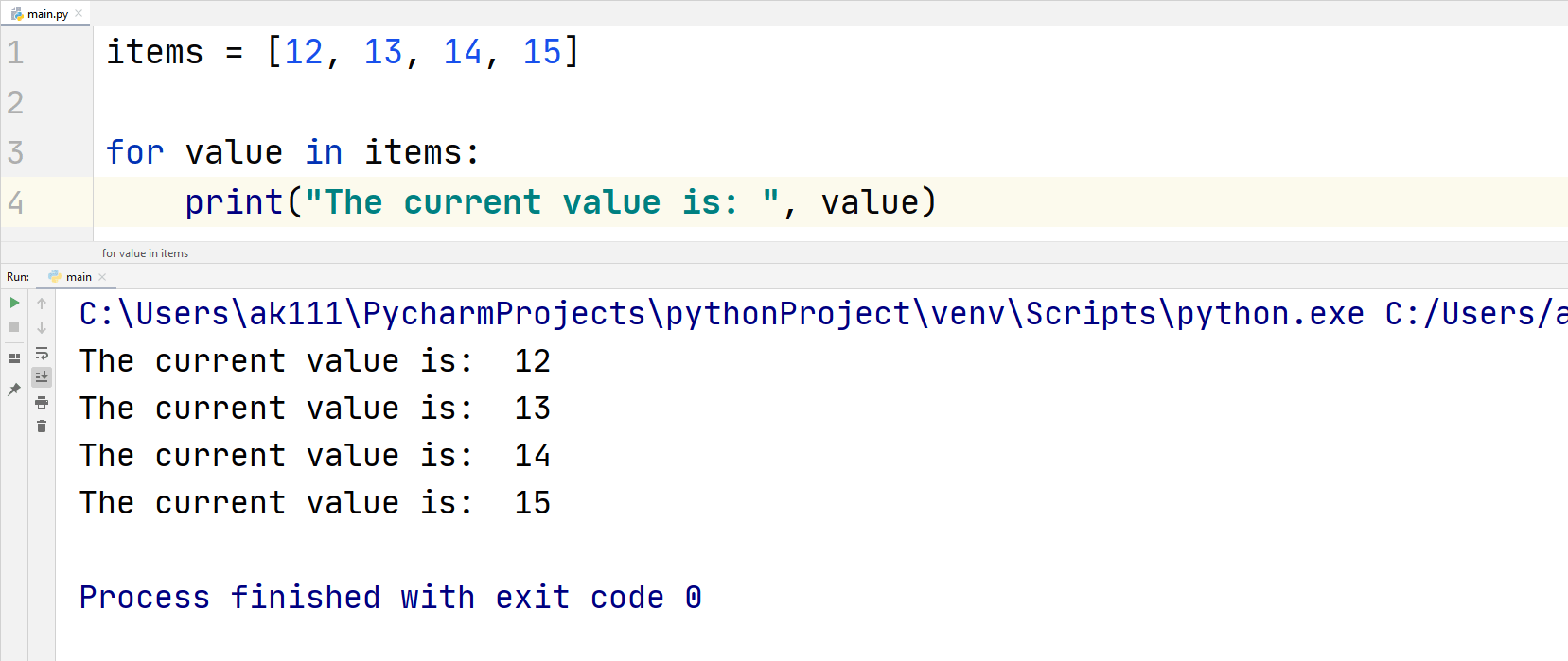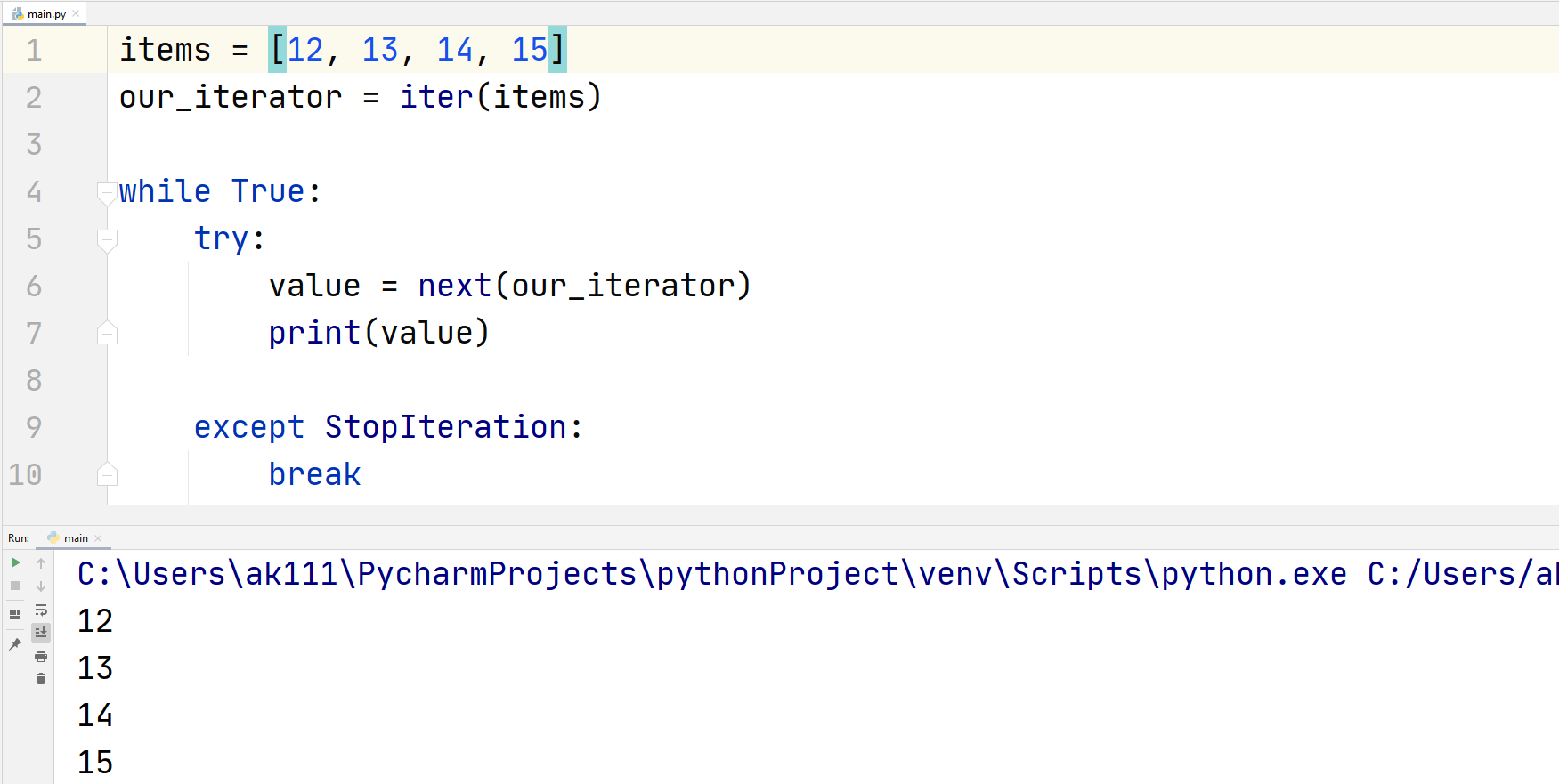# Python Iterator

## Python Iterator

An iterator in Python is a method that is used to iterate through an iterable. An iterable is basically a container for some values and iterate means to traverse through. Some examples of iterables in Python are Lists, Dictionaries, Tuples, etc.

Python is an object-oriented language and everything in Python is an object. These objects might have some methods of their own. So, the objects through which they can iterate are called iterable objects.

The method __iter()__ is the iterator method and is used to initialize an iterator. This iterator method in Python uses another method __next__ () to return the next value of the iterator.

## ExampleHow the output got carried out:

• We define an iterable object “string1” and we set its value equal to a string “Hello”
• Next, we create an iterator with the iter() method
• The next() method is used to return the next value of the iterable, as our string’s length is 5, we print the next iterator 5 times.
• If we use the next() method for the sixth time, we get an error because there are no elements left in the string to iterate through.

## For loop iterator

We can use a for loop to iterate over a dictionary or a list with the help of an index. Let us take an example of a for loop to iterate through a list.

## ExampleIn the code above, we have used the term “value” that is an iterator. The for loop can be used to iterate over any sequence and all iterables.

So how the for loop we created above is actually working, let us understand the working of the for loop first.

## ExampleAs you can see the code above is working exactly the same as a for loop. In fact, a for loop in Python is nothing but a while loop that never ends.

First, we created an iterable variable named items, then we created an iterator object our_iterator.

In the try condition of the while loop, we created a variable that stores the next iteration, and then we printed the value on the output. In the exception, we used the StopIteration statement to stop the iteration from going on forever and when we reached the end of our list, the code completed successfully.

## Python iterator Class

You can create your own iterator object using a class. The methods __iter__() and __next__() can be customized to get a desired output.

Here are some things you need to know about these two methods:

• __iter__() can be used to initialize anything and it should always return the iterator to itself
• __next__() method should return the value of the next iterator and it is generally used to create operations like addition and multiplication.

Now, with the knowledge you gained above, let us create our own custom iterators.

## ExampleWe created a method in the class that will add 5 to the initial value of the iterator and return it.

In the while loop, we used the __next__() method to print the next value of iteration 5 times.

You can technically run the iteration forever by never adding the ending condition in the while loop. These types of iterators are called infinite iterators.

## Infinite Iterators

We can use the __next__() method as much as we want. This makes the iterator an infinite iterator while using a loop you should provide the boundary conditions in the loop, otherwise, the loop would never end.

In theory, you can print infinite items using the iterator if we want to do it manually like this.

## ExampleThe print statements above can be used as many times as we want to make the iterator infinite.